## 最小生成树

### 概述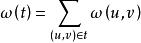（以上来自百度百科）

## Prim

### 图解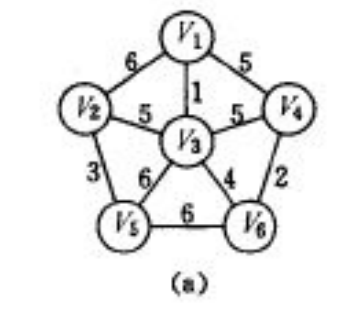dis[V2]=6,dis[V3]=1,dis[V5]=7,dis[V6]=5,dis[V4]=5；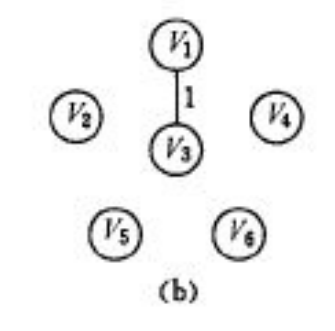dis[V2]=5,dis[V5]=6,dis[V6]=4,dis[V4]=5；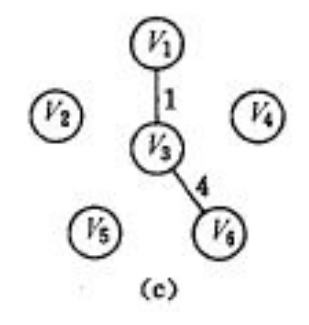dis[V2]=5,dis[V5]=6,dis[V4]=2；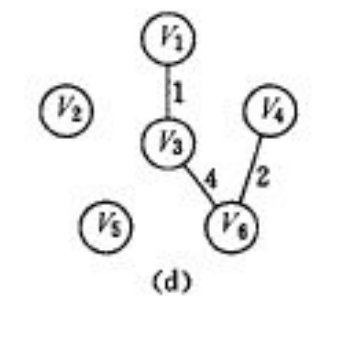dis[V2]=5,dis[V5]=6；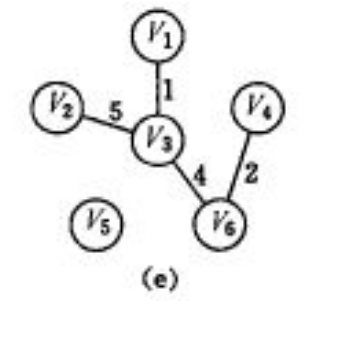dis[V5]=6；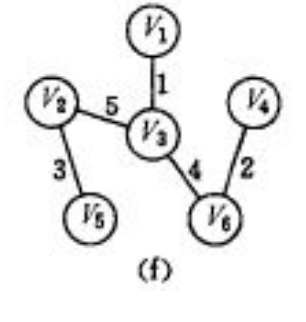### 代码（普通）

```#include<bits/stdc++.h>
using namespace std;
int f,mt,ft,dis,n,m;
void input()
{
int u,v,w;
cin>>n>>m;
memset(f,0x7f,sizeof(f));
memset(ft,0,sizeof(ft));
memset(dis,0x7f,sizeof(dis));
ft=true;
dis=0;
for(int i=1;i<=m;i++)
{
cin>>u>>v>>w;
f[i][i]=0;
f[u][v]=f[v][u]=w;
}
for(int i=1;i<=n;i++)
dis[i]=min(dis[i],f[i]);
}
void prim()
{
int k,minn;
for(int i=1;i<=n;i++)
{
k=0;minn=1e9;
for(int i=1;i<=n;i++)
{
if(!ft[i]&&dis[i]<minn)
{
k=i;
minn=dis[i];
}
}
ft[k]=true;
if(k==0) break;
mt=mt+dis[k];
for(int i=1;i<=n;i++)
if(!ft[i])
dis[i]=min(dis[i],f[i][k]);
}
}
void output()
{
cout<<mt;
}
void text1()
{
input();
prim();
output();
}
int main()
{
text1();
return 0;
}```

## Kruscal

```#include<bits/stdc++.h>
using namespace std;
int father,n,m,k,mt;
struct ed
{
int from;
int to;
int w;
}edge;
bool cmp(ed a,ed b)
{
if(a.w!=b.w)
return a.w<b.w;
}
int fat(int x)
{
if(father[x]!=x) father[x]=fat(father[x]);
return father[x];
}
void unionn(int a,int b)
{
int fa,fb;
fa=fat(a);
fb=fat(b);
if(fa!=fb) father[fa]=fb;
}
void input()
{
cin>>n>>m;
for(int i=1;i<=n;i++)
father[i]=i;
for(int i=1;i<=m;i++)
cin>>edge[i].from>>edge[i].to>>edge[i].w;
sort(edge+1,edge+m+1,cmp);
}
void kruscal()
{
for(int i=1;i<=m;i++)
{
if(fat(edge[i].from)!=fat(edge[i].to))
{
k++;
mt+=edge[i].w;
unionn(edge[i].from,edge[i].to);
}
if(k==n-1) break;
}
}
void output()
{
cout<<mt;
}
void text1()
{
input();
kruscal();
output();
}
int main()
{
text1();
return 0;
}```